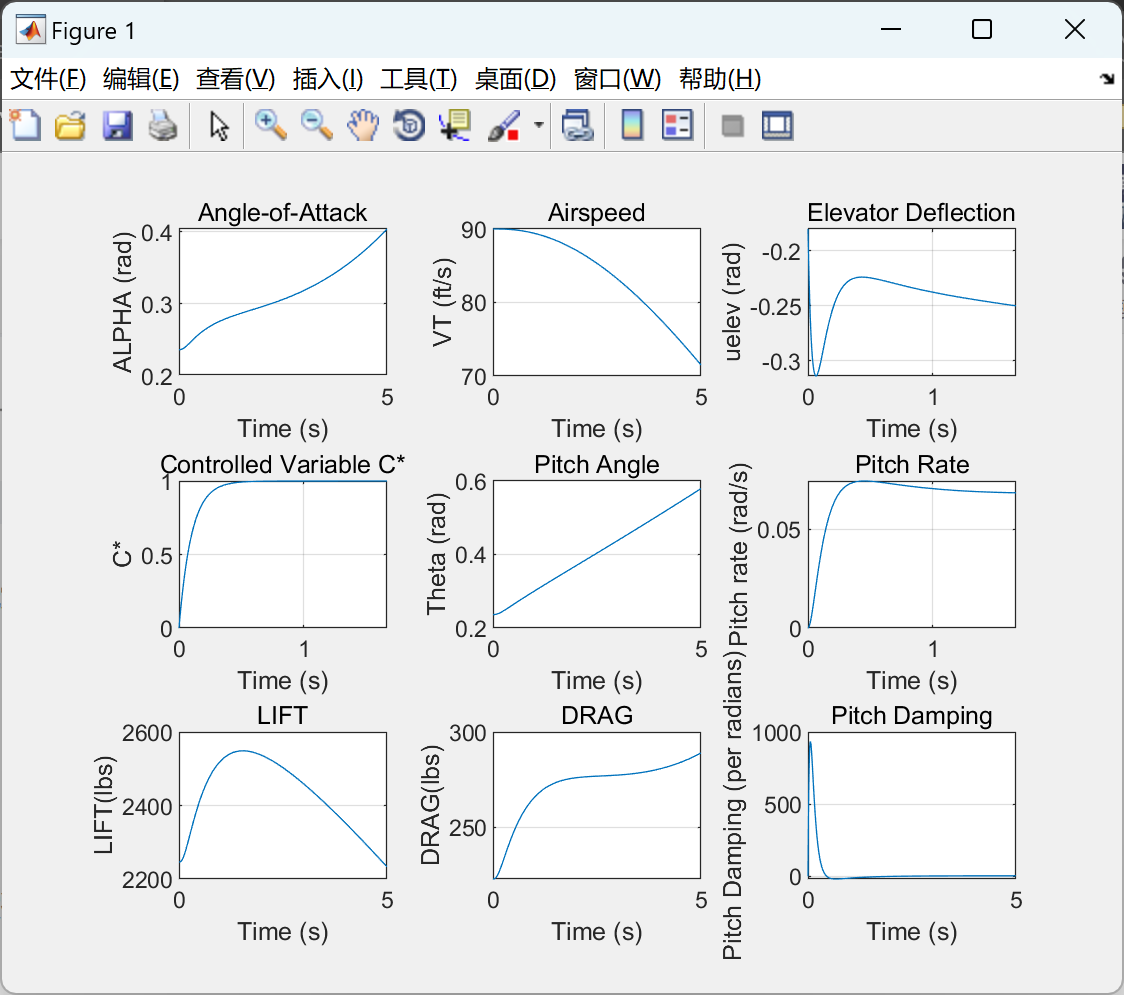# 模拟Stevens & Lewis描述的小型飞机纵向动力学的非线性动态反演控制器研究（Matlab代码实现）

+关注继续查看

## 💥1 概述

1. 系统建模：首先，你需要建立小型飞机的纵向动力学模型。这可能包括纵向运动方程、气动力模型以及控制效应模型等。这些方程需要考虑飞行器的质量、惯性、空气动力学特性等因素。你可以参考Stevens和Lewis的工作以及其他相关文献，以获取合适的模型。

2. 系统特性分析：在完成系统建模后，你可以进行系统特性分析。使用非线性系统理论，分析系统的稳定性、可控性和可观测性等特性。这些分析将有助于理解系统行为和设计控制器。

3. 动态反演控制器设计：基于系统的动力学方程和特性分析结果，设计动态反演控制器。动态反演控制器的目标是通过在线估计系统未知参数和外部扰动，实时计算出对应的控制指令。这种控制器的优势在于对系统参数变化和未建模动态的鲁棒性。

5. 实际实验：如果仿真结果令人满意，可以考虑在实际小型飞机上实施该控制器，并进行飞行试验。在实验中收集数据，并与仿真结果进行比较和分析，以验证控制器的有效性。

## 📚2 运行结果%% COMMAND INPUT
r=1;        % Reference C* demand
rdot=0;     % Reference rate

% Outputs
nz=(LIFT*cos(ALPHA)+DRAG*sin(ALPHA))/(G*MASS)-cos(THETA); % Normal acceleration [eq (2)]
nzp=nz+15*MOM/(G*IYY);  % Normal acceleration at pilot's station [eq (3)]
cstar=nzp+12.4*Q;       % Controlled C* variable [eq(4)]
y=cstar;                % Output y=h(x)

%% DYNAMIC INVERSION CONTROL INPUT
e=r-y;                          % error
Fctrl=dhdx*f;                   % F(x)
Gctrl=dhdx*g;                   % G(x)
K=10;                           % Linear control gain
uelev=(-Fctrl+rdot+K*e)/Gctrl;  % Control

%% Model State Equations
xdot=zeros(5,1);
xdot(1)=(FT*cos(ALPHA)-DRAG-WEIGHT*sin(GAMMA))/MASS;        % Airspeed rate
xdot(2)=(-FT*sin(ALPHA)-LIFT+WEIGHT*cos(GAMMA))/(MASS*VT)+Q;% AoA rate
xdot(3)=Q;                          % Pitch rate
xdot(4)=MOM/IYY;                    % Pitch acceleration
xdot(5)=-20.2*EL+20.2*uelev;        % Elevator rate

## 🎉3 参考文献

B.L. Steven & F.L. Lewis （2003） “Aircraft Flight Control & Simulation”， John Wiley （edition 2）

## 🌈4 Matlab代码实现|
3月前
|

【红外图像】利用红外图像处理技术对不同制冷剂充装的制冷系统进行性能评估（Matlab代码实现）
【红外图像】利用红外图像处理技术对不同制冷剂充装的制冷系统进行性能评估（Matlab代码实现）
26 0
|
3月前
|

【视频去噪】基于全变异正则化最小二乘反卷积是最标准的图像处理、视频去噪研究（Matlab代码实现）
【视频去噪】基于全变异正则化最小二乘反卷积是最标准的图像处理、视频去噪研究（Matlab代码实现）
66 0
|
3月前
|

47 0
|
3月前
|

62 0
|
3月前
|

【TGV 正则器的快速计算方法】通过FFT的总（广义）变化进行图像去噪（Matlab代码实现）
【TGV 正则器的快速计算方法】通过FFT的总（广义）变化进行图像去噪（Matlab代码实现）
38 0
|
3月前
|

34 0
|
3月前
|

47 0
|
3月前
|

39 0
|
3月前
|

40 0
|
4月前
|

46 0